##### Circuit Analysis For Dummies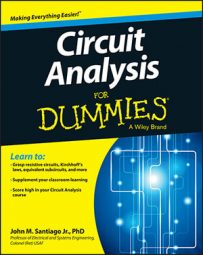The sinusoidal functions (sine and cosine) appear everywhere, and they play an important role in circuit analysis. The sinusoidal functions provide a good approximation for describing a circuit’s input and output behavior not only in electrical engineering but in many branches of science and engineering.

The sinusoidal function is periodic, meaning its graph contains a basic shape that repeats over and over indefinitely. The function goes on forever, oscillating through endless peaks and valleys in both negative and positive directions of time. Here are some key parts of the function:

• The amplitude VA defines the maximum and minimum peaks of the oscillations.

• Frequency f0 describes the number of oscillations in 1 second.

• The period T0 defines the time required to complete 1 cycle.

The period and frequency are reciprocals of each other, governed by the following mathematical relationship: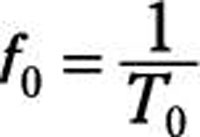Here is a cosine function you can use as the reference signal: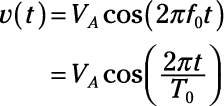You can move sinusoidal functions left or right with a time shift as well as increase or decrease the amplitude. You can also describe a sinusoidal function with a phase shift in terms of a linear combination of sine and cosine functions. Here is a cosine function and a shifted cosine function with a phase shift of π/2.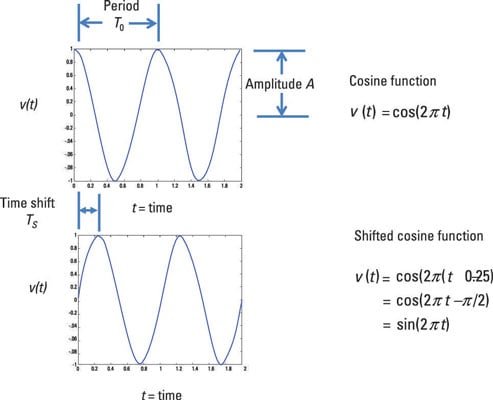## Phase shifts in a sinusoidal function

A signal that’s out of phase has been shifted left or right when compared to a reference signal:

• Right shift: When a function moves right, then the function is said to be delayed. The delayed cosine has its peak occur after the origin. A delayed signal is also said to be a lag signal because the signal arrives later than expected.

• Left shift: When the cosine function is shifted left, the shifted function is said to be advanced. The peak of the advanced signal occurs just before the origin. An advanced signal is also called a lead signal because the lead signal arrives earlier than expected.

Here are examples of unshifted, lagged, and lead cosine functions.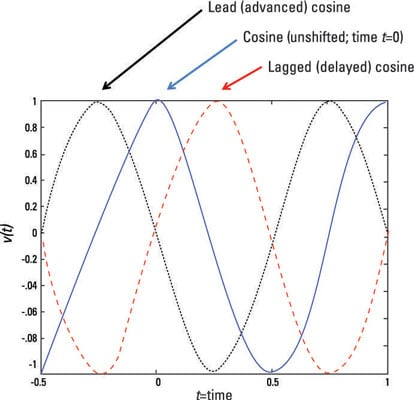To see what a phase shift looks like mathematically, first take a look at the reference signal:At t = 0, the positive peak VA serves as a reference point. To move the reference point by time shift TS, replace the t with (tTS):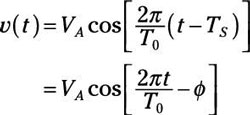where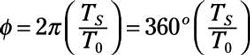The factor ϕ is the phase shift (or angle). The phase shift is the angle between t = 0 and the nearest positive peak. You can view the preceding equation as the polar representation of the sinusoid. When the phase shift is π/2, then the shifted cosine is a sine function.

Express the phase angle in radians to make sure it’s in the same units as the argument of the cosine (2πt/T0ϕ). Angles can be expressed in either radians or degrees; make sure you use the right setting on your calculator.

When you have a phase shift ϕ at the output when compared to the input, it’s usually caused by the circuit itself.

## Expand a sinusoidal function and find Fourier coefficients

The general sinusoid v(t) involves the cosine of a difference of angles. In many applications, you can expand the general sinusoid using the following trigonometric identity: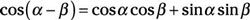Expanding the general sinusoid v(t) leads to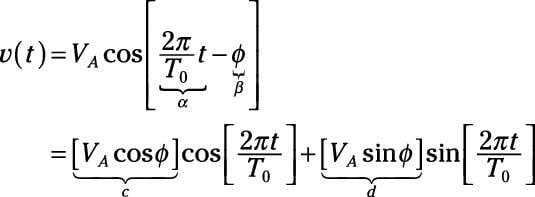The terms c and d are just special constants called Fourier coefficients. You can express the waveform as a combination of sines and cosines as follows: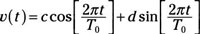The function v(t) describes a sinusoidal signal in rectangular form.

If you know your complex numbers going between polar and rectangular forms, then you can go between the two forms of the sinusoids. The Fourier coefficients c and d are related by the amplitude VA and phase ϕ: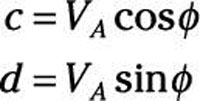If you go back to find VA and ϕ from the Fourier coefficients c and d, you wind up with these expressions: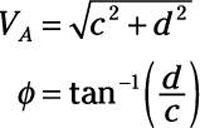The inverse tangent function on a calculator has a positive or negative 180° (or π) phase ambiguity. You can figure out the phase by looking at the signs of the Fourier coefficients c and d. Draw the points c and d on the rectangular system, where c is the x-component (or abscissa) and d is the y-component (or ordinate).

The ratio of d/c can be negative in Quadrants II and IV. Using the rectangular system helps you determine the angles when taking the arctangent, whose range is from –π/2 to π/2.

## Connect sinusoidal functions to exponentials with Euler’s formula

Euler’s formula connects trig functions with complex exponential functions. The formula states that for any real number θ, you have the following complex exponential expressions: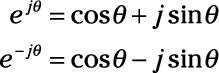The exponent jθ is an imaginary number, where j = √-1.

The imaginary number j is the same as the number i from your math classes, but all the cool people use j for imaginary numbers because i stands for current.

You can add and subtract the two preceding equations to get the following relationships: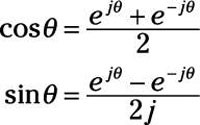These equations say that the cosine and sine functions are built as a combination of complex exponentials. The complex exponentials play an important role when you’re analyzing complex circuits that have storage devices such as capacitors and inductors.